# Chain rule

## Summary and examples

When we want to differentiate a function we can use the table of derivatives of standard functions, sometimes in combination with the product and quotient rule. Sometimes this is not possible, see the following example:This function can not be differentiated easily by merely using the table and product or quotient rules. In such cases we can use the chain rule.

There are both a formal and informal version of the chain rule. We explain the formal way by the following example.

##### Example 1

Differentiate:This function is not in the table of derivatives of standard functions and the product rule is not applicable.

We apply the transformation: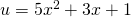that is to say, we switch to another variable. Then we get: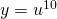Now we apply the following rule (the chain rule):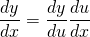and get: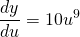and: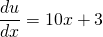And thus: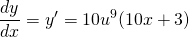We want the result in only the variableand get: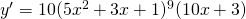##### Example 2

Differentiate: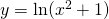If we define: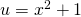we get: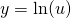and this function is in our table with standard functions. Applying the chain rule yields: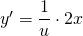and, back to: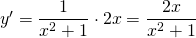The informal method works as follows. We look again at the previous functions. If we want to differentiate: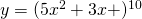we could naively apply the table: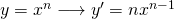which would have resulted in: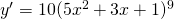but this is wrong. To get the correct answer we have to multiply this preliminary result by the derivative of the function that took the place ofin the function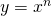. In this case we have to multiply by the derivative of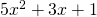which is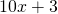. Then the result is: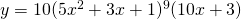In the second example the 'naive differentiator' would have got as the derivative: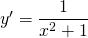but this is not the right answer. Again, to get the correct derivative, we have to multiply this result by the derivative of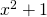which is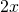. The correct answer is: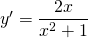A few more examples.

##### Example 3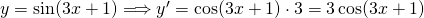In this example we differentiate the standard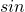-function and multiply the result by the derivative of.

##### Example 4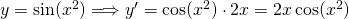Again the-function is the standard function and the derivative is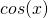. We have to multiply this result by the derivative of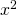.

##### Example 5

Differentiate: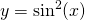In this example we need to differentiate a square of the function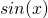. This means that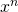is the standard function and thatis in place of.

First we differentiate: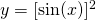and multiply the result by the derivative of.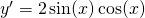Note that the chain rule is an extra method to existing methods: the derivatives of standard functions and the product and quotient rule. We can combine these methods to determine a derivative, see the following example.

##### Example 6

Differentiate: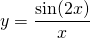In this case the numerator is: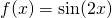and the denominator is:and thus we have: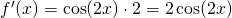(chain rule)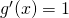(table)

and thus the result is: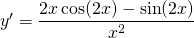0